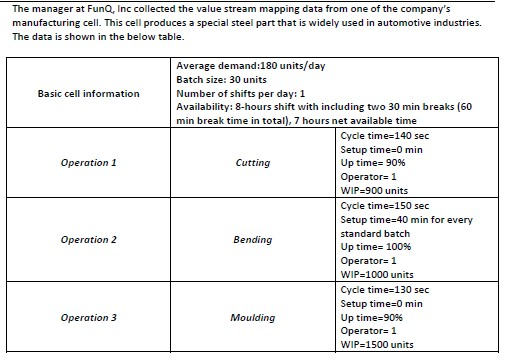# Answered! Based on the given data answer the following questions: i. What is the Takt time?…Based on the given data answer the following questions:

Don't use plagiarized sources. Get Your Custom Essay on
Answered! Based on the given data answer the following questions: i. What is the Takt time?…
GET AN ESSAY WRITTEN FOR YOU FROM AS LOW AS \$13/PAGE

i. What is the Takt time?

ii. Calculate the actual cycle times for each operation.

iii. Calculate the production lead time.

The manager at FunQ, nc collected the value stream mapping data from one of the company’s manufacturing cell. This cell produces a special steel part that is widely used in automotive industries. The data is shown in the below table. Average demand 180 units/day Batch size: 30 units Number of shifts per day: 1 Basic cel nformation Availability: 8-hours shift with including two 30 min breaks (60 min break time in total), 7 hours net available time Cycle time-140 sec Setup time 0 min operation 1 Cutting Uptime 9096 operator 1 WIP. 900 units Cycle time-150 sec setup time 40 min for every standard batch operation 2 Bending Uptime 100% operator 1 WIP-1000 units Cycle time-130 sec Setup time 0 min operation 3 Moulding Up time-9096 Operator 1 WIP-1500 units

i. takt time = Net available time / average demand per day = 7*60/180 = 2.33 minutes = 140 sec

ii.

Actual cycle time for Cutting operation = Cycle time / Uptime = (140 + 0) / 90% = 155.6 sec

Actual cycle time for Bending operation = (150 + 40*60/30) / 100% = 230 sec

Actual cycle time for Moulding operation = (130 + 0) / 90% = 144.4 sec

iii. Bottleneck operation is Bending. Throughput of the whole system is equal to the throughput of the bottleneck operation. Therefore, throughput = 3600/230 = 15.65 per hour.